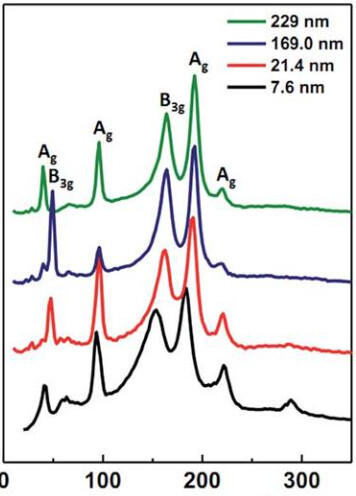# Tabular regression and classification?

Does anybody have any advice on how to setup a tabular databunch and learner for a regression and classification problem?

The `TabularPandas` class `y_block` argument won’t take a list of `RegressionBlock()` and `MultiCategoryBlock`. It’s looking for a single TransformBlock and is giving an error that the list doesn’t have a ‘type_tfms’ attribute. This is what I’m trying right now

``````to = TabularPandas(df, procs = [Categorify, Normalize, FillMissing],
y_names = targets,
y_block = (RegressionBlock(n_out = 15), MultiCategoryBlock()),
cat_names = cat_names,
cont_names = cont_names,
splits = ColSplitter('is_valid')(df),
)
``````

which gives the following error

``````AttributeError                            Traceback (most recent call last)
/tmp/ipykernel_24346/3903240160.py in <module>
----> 1 to = TabularPandas(df, procs = [Categorify, Normalize, FillMissing],
2                    y_names = targets,
3                    y_block = [RegressionBlock(n_out = 15), MultiCategoryBlock()],
4                    cat_names = cat_names,
5                    cont_names = cont_names,

~/miniconda3/envs/emp_path/lib/python3.9/site-packages/fastai/tabular/core.py in __init__(self, df, procs, cat_names, cont_names, y_names, y_block, splits, do_setup, device, inplace, reduce_memory)
161         if y_block is not None and do_setup:
162             if callable(y_block): y_block = y_block()
--> 163             procs = L(procs) + y_block.type_tfms
164         self.cat_names,self.cont_names,self.procs = L(cat_names),L(cont_names),Pipeline(procs)
165         self.split = len(df) if splits is None else len(splits)

AttributeError: 'list' object has no attribute 'type_tfms'
``````

To give some more details on my dataset I’ve got tabular dataset representing a spectroscopy measurement which I’d like to predict the location of peaks (regression targets) and the classification of that peak for each example. For example (not my actual problem, but same idea) I’d like to train the model to take in the spectra and identify the location and classification of the peaks as shown in the figure here.